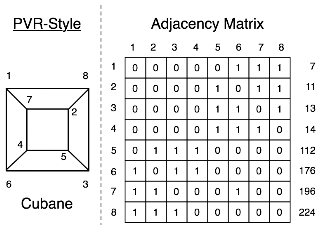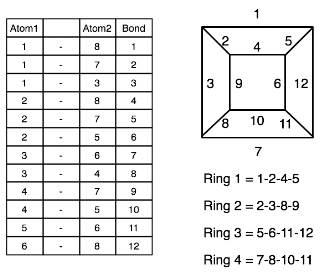Skip to main content

### On Canonical Numberings

So, after reading* this (2005) paper : "On Canonical Numbering of Carbon Atoms in Fullerenes : C60 Buckminsterfullerene" (link) I made some pictures to illustrate the difference between it and the numbering scheme used for SMILES (as described here). Er, which is used in the CDK.

Anyway, the point is that the scheme used by Plavšić, Vukičević, and Randić (or PVR as I will refer to them, I hope they don't mind!) numbers the atoms in a way that produces an adjacency matrix with a particular property. If you consider the rows of the matrix to be binary numbers, then the set of numbers is the smallest possible. So, for example:The structure on the left is cubane, with its adjacency matrix on the right. The column on the far right shows the rows of the matrix in base 10. They are clearly in order. Now what happens for the SMILES? Well:Here, the rows are neither in order (I'm not sure from their paper whether the ordering is an expected outcome for all structures, nor have I checked...) nor is their sum less than for PVR scheme - 765 vs 753.

Of course, the PVR labelling would be useless for generating SMILES for cubane since there is no way to get a path from it. Indeed, the labels are designed to be maximally unfriendly by pairing the highest with the lowest.

Furthermore their scheme goes on to label bonds and rings:Which also look quite random; or, as they say :
"... we admit that the final labels ... do not appear »orderly« but one has to recognise that there is no »simple« labelling in [fullerenes] that will appear simple"
which makes sense. Obviously, not for cubane here, but for C60/C70 and so on it does.
*(probably because the name follows the "On X" paper naming scheme)

### How many isomers of C4H11N are there?

One of the most popular queries that lands people at this blog is about the isomers of C4H11N - which I suspect may be some kind of organic chemistry question on student homework. In any case, this post will describe how to find all members of a small space like this by hand rather than using software.

Firstly, lets connect all the hydrogens to the heavy atoms (C and N, in this case). For example:

Now eleven hydrogens can be distributed among these five heavy atoms in various ways. In fact this is the problem of partitioning a number into a list of other numbers which I've talked about before. These partitions and (possible) fragment lists are shown here:

One thing to notice is that all partitions have to have 5 parts - even if one of those parts is 0. That's not strictly a partition anymore, but never mind. The other important point is that some of the partitions lead to multiple fragment lists - [3, 3, 2, 2, 1] could have a CH+NH2 or an NH+CH2.

The final step is to connect u…

### The Gale-Ryser Theorem

This is a small aside. While reading a paper by Grüner, Laue, and Meringer on generation by homomorphism they mentioned the Gale-Ryser (GR) theorem. As it turns out, this is a nice small theorem closely related to the better known Erdős-Gallai (EG).

So, GR says that given two partitions of an integer (p and q) there exists a (0, 1) matrixA iff p*dominatesq such that the row sum vector r(A) = p and the column sum vector c(A) = q.

As with most mathematics, that's quite terse and full of terminology like 'dominates' : but it's relatively simple. Here is an example:

The partitions p and q are at the top left, they both sum to 10. Next, p is transposed to get p* = [5, 4, 1] and this is compared to q at the bottom left. Since the sum at each point in the sequence is greater (or equal) for p* than q, the former dominates. One possible matrix is at the top left with the row sum vector to the right, and the column sum vector below.

Finally, the matrix can be interpreted as a bi…

### Havel-Hakimi Algorithm for Generating Graphs from Degree Sequences

A degree sequence is an ordered list of degrees for the vertices of a graph. For example, here are some graphs and their degree sequences:

Clearly, each graph has only one degree sequence, but the reverse is not true - one degree sequence can correspond to many graphs. Finally, an ordered sequence of numbers (d1 >= d2 >= ... >= dn > 0) may not be the degree sequence of a graph - in other words, it is not graphical.

The Havel-Hakimi (HH) theorem gives us a way to test a degree sequence to see if it is graphical or not. As a side-effect, a graph is produced that realises the sequence. Note that it only produces one graph, not all of them. It proceeds by attaching the first vertex of highest degree to the next set of high-degree vertices. If there are none left to attach to, it has either used up all the sequence to produce a graph, or the sequence was not graphical.

The image above shows the HH algorithm at work on the sequence [3, 3, 2, 2, 1, 1]. Unfortunately, this produce…